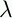September 22, 1999 Perl and the Lambda Calculus Slide #36

# Applicative Order Solution

• Instead of IF c x y, use

```        (IF cq.xq.y)
```
•here delays evaluation

• Later, the result of the IF will be one or the other of these functions

• Apply it to a dummy argument to yield the real result

• IF can take care of applying to the dummy argument if we want:

```        IF =cxy.((c x y) Q)
```
• Q can be chosen arbitrarily, since it's thrown away

• We'll take Q =i.i from now on

 NextCopyright © 1999 M-J. Dominus